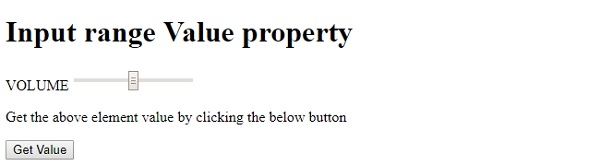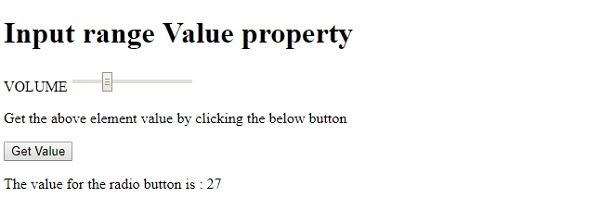HTML DOM Input Range value property

HTMLWeb DevelopmentFront End Technology

The HTML DOM Input range value property is associated with the input element having type=”range” and the value attribute. It is used to return the value of slider control value attribute or to set it. The value attribute can be the default value or the value set by dragging the slider.

Syntax

Following is the syntax for −

Setting the value property −

rangeObject.value = text;

Here, text is used for specifying the value for the range slider control.

Example

Let us look at an example for the input range value property −

Live Demo

<!DOCTYPE html>
<html>
<body>
<h1>Input range Value property</h1>
<form>
VOLUME <input type="range" id="RANGE1" name="VOL">
</form>
<p>Get the above element value by clicking the below button</p>
<button onclick="getValue()">Get Value</button>
<p id="Sample"></p>
<script>
function getValue() {
var t = document.getElementById("RANGE1").value;
document.getElementById("Sample").innerHTML = "The value for the radio button is : "+t;
}
</script>
</body>
</html>

Output

This will produce the following output −On clicking the “Get Value” button −In the above example −

We have created an input field contained inside a form having type=“range”, id=“RANGE1”, name=“VOL” −

<form>
VOLUME <input type="range" id="RANGE1" name="VOL">
<form>

We have then created a button “Get Value” that will execute the getValue() method when clicked by the user −

<button type="button" onclick="getValue()">Get Value</button>

The getValue() method gets the input element using the getElementById() method and assigns its “value” attribute value to variable t. This variable is then displayed in the paragraph with id “Sample” using its innerHTML property −

function getValue() {
var t = document.getElementById(“RANGE1").value;
document.getElementById("Sample").innerHTML = "The value for the input field is : "+t;
}